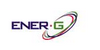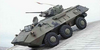### APXIn complexity theory the class APX (an abbreviation of `approximable`) is the set of NP optimization problems that allow polynomial-time approximation algorithms with approximation ratio bounded by a constant (or constant-factor approximation algorithms for short). In simple terms, problems in this class have efficient algorithms that can find a.....
Found on http://en.wikipedia.org/wiki/APX

### APXAmsterdam Power Exchange
Found on http://www.energ.co.uk/Glossary

### APXSeries of turret designs [FR]
Found on http://www.jedsite.info/index.html
No exact match found